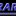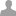## Interfacing 5V device to a 3.3V Analoginput

The mbed AnalogInput has a maximum input voltage of 3.3V. I have a 5V device that I would like to interface to it, what should I do? (option #1) using a voltage divider with two 1K Ohm resistors. (option #2) how about using an op-amp?

Anybody has a better solution?

Here is what I am trying to do: I have a Allegro A3516 hall sensor has a range from 0V to 5V output. Which represent -800Gauss to +800Gauss. When it is at 2.5V, it is 0 Gauss.

A voltage divider is the easiest solution. You may want to use different values than 2 resistors of 1K. These values result in a maximum voltage of 2.5V, meaning you dont use the full range of the AD converter and loose some resolution. You may also want to use larger values to reduce the load on the sensor, but not too large to avoid noise. Op-amps need separate supply, possibly negative, to work. They may be used when your sensor signal needs amplification, buffering or filtering. They may also be used to shift the voltage range. For example when you only want the positive gauss measurement and decide to modify the 2.5V-5V into a voltage range between 0-3.3V.

If this is an experiment rather than a production application you could try powering the sensor from 3.3v instead of 5v. The output is proportional to supply, so if it works it should idle at 1.65v and give 0 or 3.3v for +/- 800gauss, though it would be out of specification (YMMV).

Allegro do make a 3.3v sensor now.

With these devices the sensor and MBED should be powered from the same supply. The sensor produces an output proportional to the supply voltage (ratiometric), and since the MBED measures relative to supply the resulting digital reading is independant of variations in supply.

Incidentally if it won't run from 3.3v then note that a 1.5:1 divider such as 10k series, 20k to ground should scale 5v down to very nearly 3.3v.

If you use 47K, and 91K it scles prfect.

Just multiply by 5.0 not 3.3 to give volts.

To achive correct bipolar range, you will need some analoge electronics,

Probably subtract USB/2 volts .... Caution not actualy 5 volts guarenteed.

I highly recommend the TXB0104/08 from TI. http://octopart.com/partsearch#search/requestData&q=TXB0104

####ohararp@... wrote:

I highly recommend the TXB0104/08 from TI. http://octopart.com/partsearch#search/requestData&q=TXB0104

Analog application: digital translators won't scale a voltage down in proportion.

For what its worth the TXB/S parts aren't going to solve every digital case. The TXB parts look only suited to CMOS-to-CMOS interfacing. Anything that loads the pin such as a termination, pull-up or transistor, is likely to lead to poor results.

I would put 3 diodes forward-biased, in series to achieve a near 3.3V from 5V, assuming that the 5V source is trusted. If not I would add a Zener + a current limiting transistor at the output (in parallel to the 3.3V load).

####Narendran Kumaragurunathan wrote:

I would put 3 diodes forward-biased, in series to achieve a near 3.3V from 5V, assuming that the 5V source is trusted.

Series diodes are not a good solution in this case. You want to measure down to 0 volts since that represents a valid input value. The series diodes have a threshold of 1.8 volts, meaning you dont get any input on the ADC in the low range. Diodes are also not linear.

####Narendran Kumaragurunathan wrote:

I would put 3 diodes forward-biased, in series to achieve a near 3.3V from 5V, assuming that the 5V source is trusted.

Series diodes are not a good solution in this case. You want to measure down to 0 volts since that represents a valid input value. The series diodes have a threshold of 1.8 volts, meaning you dont get any input on the ADC in the low range. Diodes are also not linear.

Yes, it depends on the load (& the voltage variation the load can accomodate). If accurate voltage is required at the output, we can use the (parallel) zener solution mentioned before. One more could be adding a reverse-biased 1.6v Zener + an optional small resistor (< 100ohms, note that resistor can add a small variation to the o/p voltage) in series to the 3.3v load.

Ceri recommended 47K and 91K, which I agree looks like a great fit.

Robert said his sensor is on a 5v supply, and the signal is likely proportional to that supply. So it has the potential for error based on that supply (is it 5.000v or somewhat higher or lower). So, another pair of resistors, used to measure the 5v supply itself may be necessary to extract the most accurate signal. These need to be matched, or precision resistors to avoid introducing more errors.

In this situation I too would not recommend the diodes; near-zero sensing, diode variations and temperature sensitivity could ruin the accuracy of the measurement.

Lastly, filter the analog input as heavily as possible, because the mbed analog input is often noisy. This filtering could be with capacitors at the analog inputs, software, or both. Depending on how fast your input signal may change, there will be some compromise here.